# Nearest greater to right

Category: Data Structures
Tags: #datastructures#stack

Write a program to find the nearest greater element or next greater to the right of each element in an array.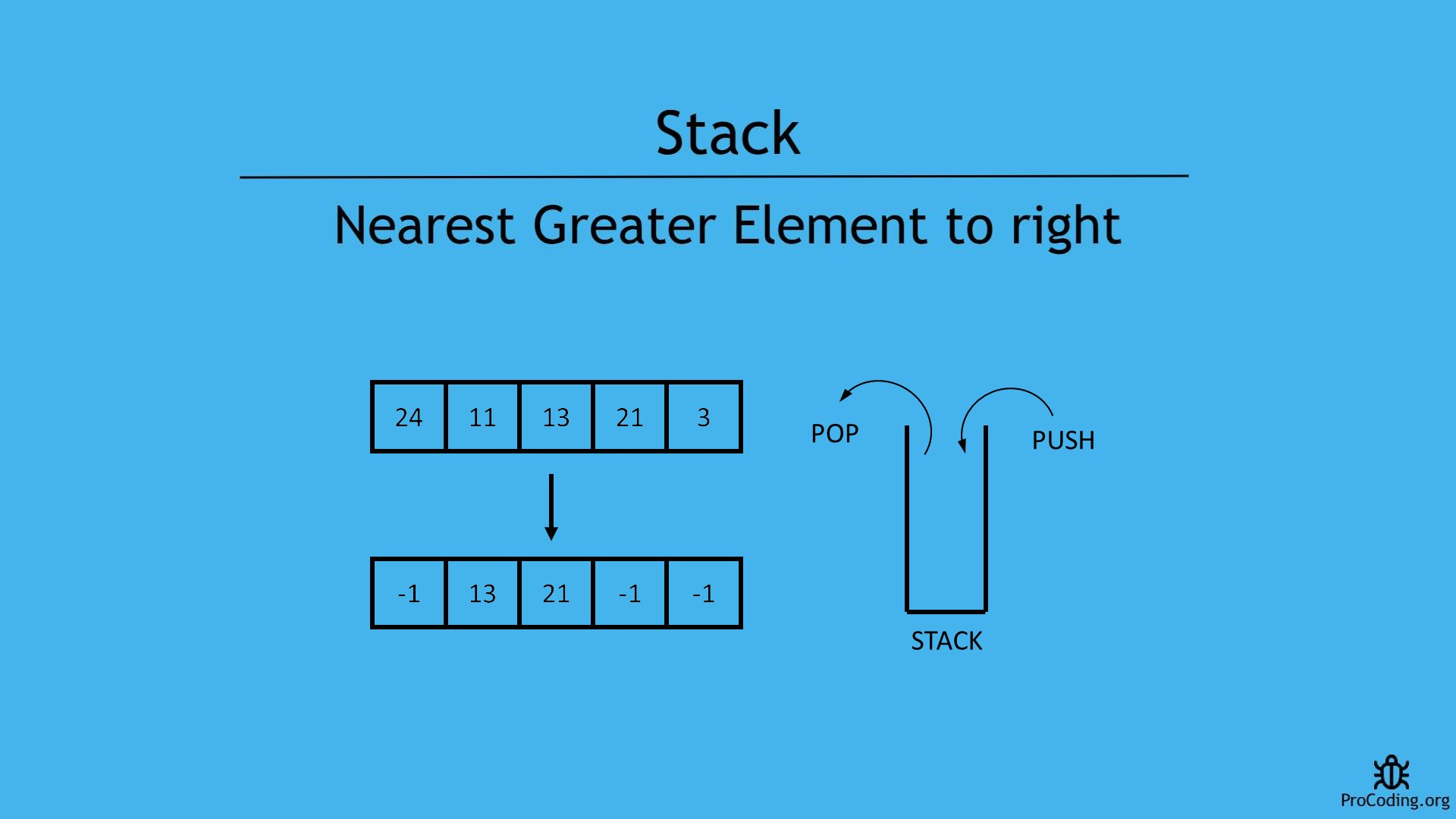### Problem

In case of this problem, an array is given and we have to find first greater element to the right of current element. Similalrly, find the greater elements for each element in the array and if greater element is not available then return a default value based on the problem.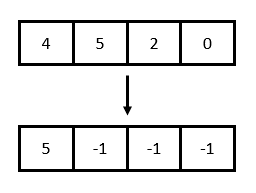Example -

``````Input: [4, 5, 2, 0]
Output: [5, -1, -1, -1]

Input: [13, 7, 6, 12]
Output: [-1, 12, 12, -1]
``````

Few points to consider:

1. If the array is sorted in decreasing order, next greater element will always be -1.
2. For the right most element, next greater element will always be -1.

We will see two ways to solve the problems

### Brute-force approach (using nested loops)

In this approach we will use two loops. Outer loop will iterate over all the array items and inner loop will iterate over all the next elements of currently pointed by outer loop. Inner loop will check, if any greater element will be found then loop will be terminated and if greater element is not found then `-1` will be added as result.

Python
``````def nearestGreaterToRight(arr):
result = []
for i in range(0, len(arr)):
for j in range(i+1, len(arr)):
if arr[i] < arr[j]:
result.append(arr[j])
break
else:
result.append(-1)

return result

arr = [11, 13, 21, 3]
print(nearestGreaterToRight(arr))
``````
JavaScript
``````function nearestGreaterToRight(arr) {
let result = [];

for (let i = 0; i < arr.length; i++) {
let flag = false;
for (let j = i + 1; j < arr.length; j++) {
if (arr[i] < arr[j]) {
result.push(arr[j]);
flag = true;
break;
}
}
if (!flag) {
result.push(-1);
}
}

return result;
}

let arr = [11, 13, 21, 3];
console.log(nearestGreaterToRight(arr));
``````

Output

``````[13, 21, -1, -1]
``````

Although, we have solved this problem using loops but this is not an optimized solution.
Because time complexity of this solution is O(n^2).

### Using stack

Algorithm

``````NearestGreaterToRight(A)
1. for i = A.length to 0
2.   if stack is empty
4.     stack.push(A[i])
5.   else if stack is empty
6.     while (stack is not empty AND A[i] > top of stack)
7.       stack.pop()
8.     if stack is empty
10.    else
12.    stack.push(A[i])
13. return reverse of result
``````

In this approach, we are using a stack.

We have to start traversing elements of the given array from the end i.e., rightmost element and we will be putting the highest element in the stack and whenever we get another highest element then pop the stack and push the new element. Basically, we will be using a stack to keep values available to the right side and which are the greater ones.

If the stack is empty the greater element to right will be `-1` otherwise top of stack will be the highest element. At the end we have to reverse the result array because we are working on the input array from the opposite side.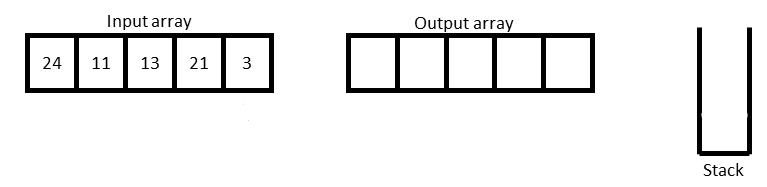Initially, input array will be given and an output array with stack will be created.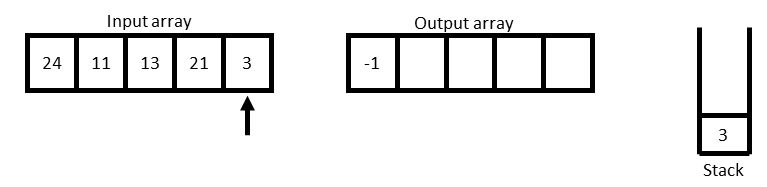Start traversing of array from the right side and for the rightmost element nearest greater to right will be `-1` and put the value from the input array into the stack for further comparision.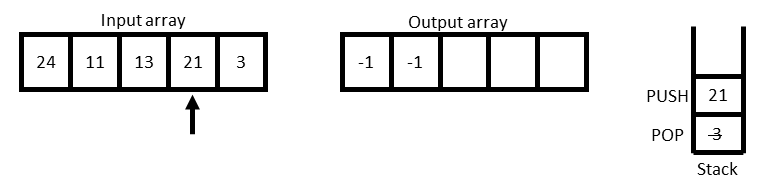For 21, stack is not empty so we have to check the top most value if it greater than 21 or not. Here we observe that 3 not greater than 21 so pop out 3 and now stack is empty so nearest greater element will be `-1` and push 21 into the stack.For 13, there is already an element in the stack which is greater than 13 so that will be the inserted into the output array and 13 will be pushed into the stack.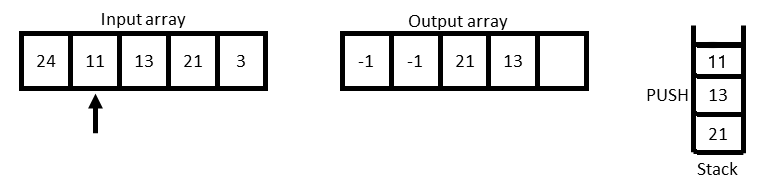For 11, the top of stack is occupied by 13 which is greater than 11 so 13 will be inserted into the result array and 11 will pe pushed into the stack.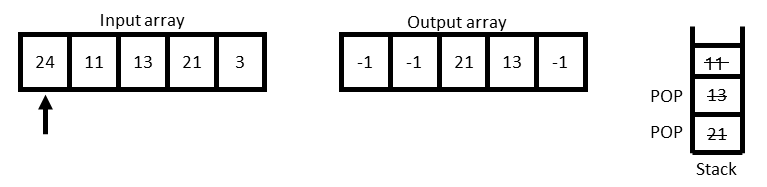For 24, all the values available in the stack are less than 24 so all the vlaues will be popped off one by one and at the end stack will be empty so `-1` will be inserted into the output array.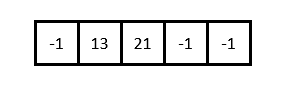At the end, reverse the output array because we have started the traversal of array from right end and this will be the result.

#### Program

Python
``````class Stack:
def __init__(self):
self.stack = []

def isEmpty(self):
return len(self.stack) == 0

def push(self, num):
self.stack.append(num)

def pop(self):
if self.isEmpty():
raise Exception('Stack Underflow')
return self.stack.pop()

def peek(self):
if self.isEmpty():
return None
return self.stack[-1]

def nearestGreaterToRight(arr):
stack = Stack()
result = []

for i in range(len(arr)-1, -1, -1):
if stack.isEmpty():
result.append(-1)
stack.push(arr[i])
elif not stack.isEmpty():
while(not stack.isEmpty() and arr[i] > stack.peek()):
stack.pop()
if stack.isEmpty():
result.append(-1)
else:
result.append(stack.peek())
stack.push(arr[i])

result.reverse()
return result

arr = [11, 13, 21, 3]
print(nearestGreaterToRight(arr))
``````
JavaScript
``````class Stack {
constructor() {
this.stack = [];
}

isEmpty() {
return this.stack.length === 0;
}

push(num) {
this.stack.push(num);
}

pop() {
if (this.isEmpty()) {
throw "Stack Underflow";
}
return this.stack.pop();
}

peek() {
if (this.isEmpty()) {
return null;
}
return this.stack[this.stack.length - 1];
}
}

function nearestGreaterToRight(arr) {
const stack = new Stack();
let result = [];

for (let i = arr.length - 1; i >= 0; i--) {
if (stack.isEmpty()) {
result.push(-1);
stack.push(arr[i]);
} else if (!stack.isEmpty()) {
while (!stack.isEmpty() && arr[i] > stack.peek()) {
stack.pop();
}
if (stack.isEmpty()) {
result.push(-1);
} else {
result.push(stack.peek());
}
stack.push(arr[i]);
}
}

result.reverse();
return result;
}

arr = [11, 13, 21, 3];
console.log(nearestGreaterToRight(arr));
``````

Output

``````[13, 21, -1, -1]
``````